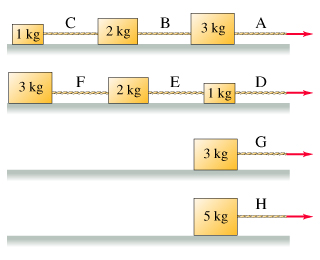# Problem: Below are eight crates of different mass.  The crates are attached to massless ropes, as indicated in the picture, where the ropes are marked by letters.  Each crate is being pulled to the right at the same constant speed.  The coefficient of kinetic friction between each crate and the surface on which it slides is the same for all eight crates.Rank the ropes on the basis of the force each exerts on the crate immediately to its left.Rank from largest to smallest. To rank items as equivalent, overlap them.

###### FREE Expert Solution

Kinetic coefficient of friction:

$\overline{){{\mathbf{f}}}_{{\mathbf{k}}}{\mathbf{=}}{{\mathbf{\mu }}}_{{\mathbf{k}}}{\mathbf{N}}}$

Newton's second law:

$\overline{){\mathbf{F}}{\mathbf{=}}{\mathbf{m}}{\mathbf{a}}}$

Let the y-axis point vertically upward and the x-axis horizontally.

Applying Newton's second law vertically — the blocks are at rest with respect to the y-axis:

ΣFy = N - mg = 0

N = mg

Thus:

fk = μkN = μkmg

Applying Newton's second law horizontally — the blocks are moving with constant velocity, a = 0:

ΣFx,C = TC - fk,C = 0

TC = fk,C = μkmCg = μk(1)(9.8) = 9.8μk

ΣFx,B = TB - TC - fk,C = 0

90% (308 ratings)###### Problem Details

Below are eight crates of different mass.  The crates are attached to massless ropes, as indicated in the picture, where the ropes are marked by letters.  Each crate is being pulled to the right at the same constant speed.  The coefficient of kinetic friction between each crate and the surface on which it slides is the same for all eight crates.Rank the ropes on the basis of the force each exerts on the crate immediately to its left.

Rank from largest to smallest. To rank items as equivalent, overlap them.